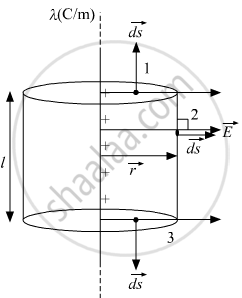# State Gauss's law on electrostatics and drive an expression for the electric field due to a long straight thin uniformly charged wire (linear charge density λ) at a point lying - Physics

State Gauss's law on electrostatics and drive expression for the electric field due to a long straight thin uniformly charged wire (linear charge density λ) at a point lying at a distance r from the wire.

#### Solution

Gauss' Law states that the net electric flux through any closed surface is equal to 1/epsilon_0 times the net electric charge within that closed surface.

oint  vec" E".d vec" s" = (q_(enclosed))/epsilon_oIn the diagram, we have taken a  cylindrical gaussian surface of radius = r and length = l.
The net charge enclosed inside the gaussian surface q_(enclosed) = lambdal
By symmetry, we can say that the Electric field will be in radially outward direction.

According to gauss' law,

oint  vec"E".d  vec"s" = q_(enclosed)/epsilon_o

int_1 vec"E" .d  vec"s" + int_2  vec"E" .d  vec"s" + int_3  vec"E". d  vec"s" = (lambdal)/epsilon_o

int_1  vec"E". d  vec"s"  &  int_3  vec"E". d  vec"s"  "are zero", "Since"  vec"E"  "is perpendicular to"  d  vec"s"

int_2  vec"E" . d  vec"s" = (lambdal)/epsilon_o

"at"  2,  vec"E" and d  vec"s"  "are in the same direction, we can write"

E.2pirl = (lambdal)/epsilon_o

E = lambda/(2piepsilon_o r)

Concept: Gauss’s Law
Is there an error in this question or solution?
2019-2020 (February) Delhi (Set 2)

Share